You are currently offline. Some features of the site may not work correctly.

# Minkowski's question mark function

Known as: Conway box function, %3F function, %3F(x)
In mathematics, the Minkowski question mark function (or the slippery devil's staircase), denoted by ?(x), is a function possessing various unusual… Expand
Wikipedia

## Papers overview

Semantic Scholar uses AI to extract papers important to this topic.
2017
2017
1 : We use properties of the Stern Sequence for numerical computations of moments 1 0 t n d?(t) associated to Minkowski's… Expand
•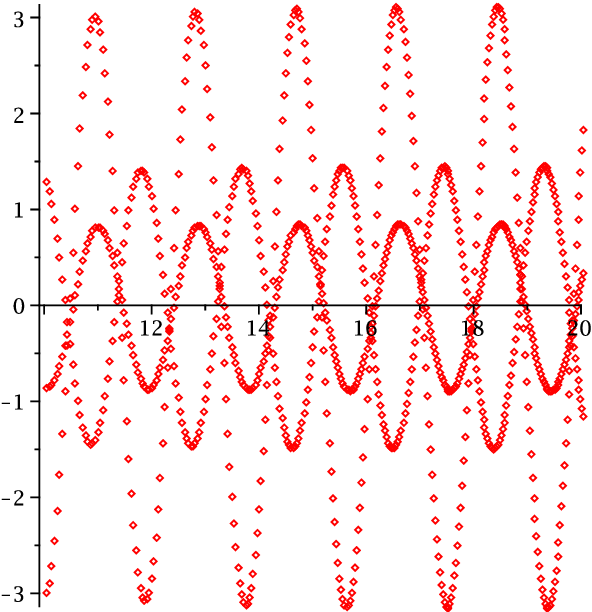2017
2017
• G. Mantica
• J. Approx. Theory
• 2017
• Corpus ID: 205707449
Minkowski's question mark function is the distribution function of a singular continuous measure: we study this measure from the… Expand
•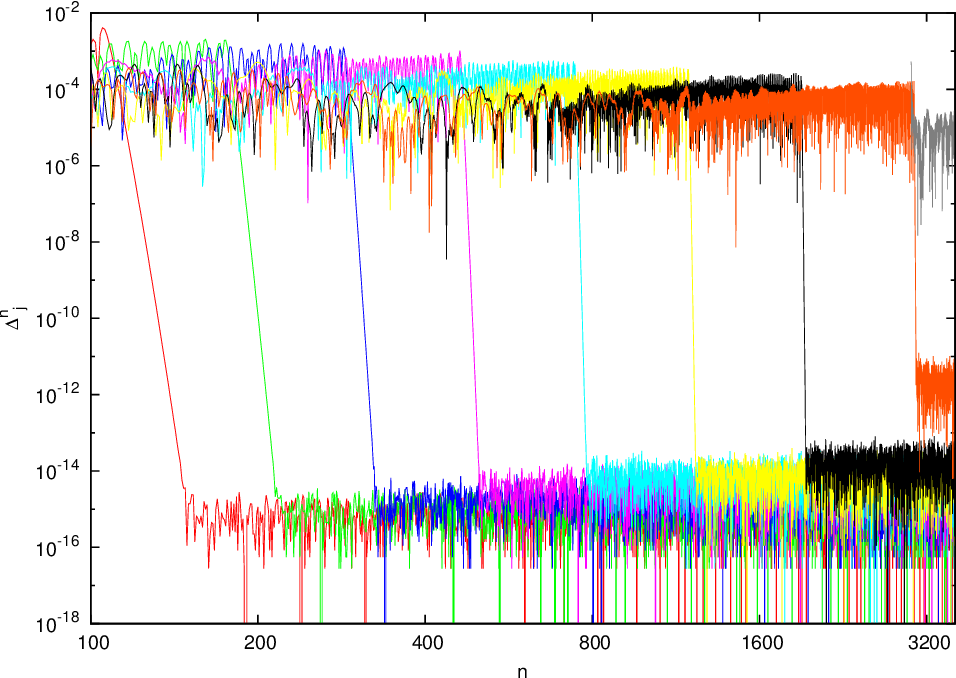•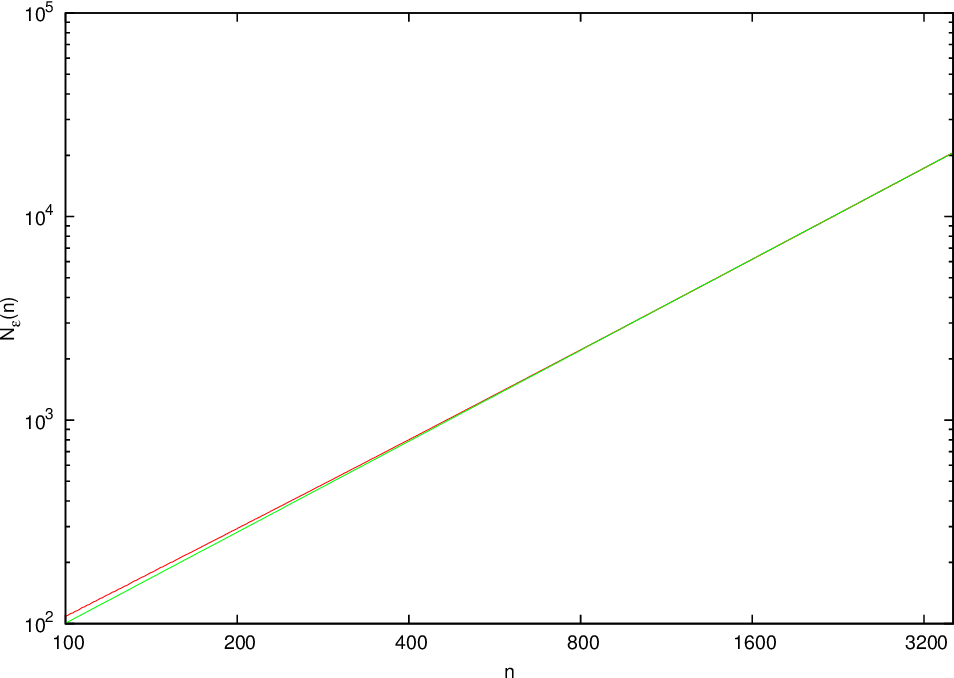•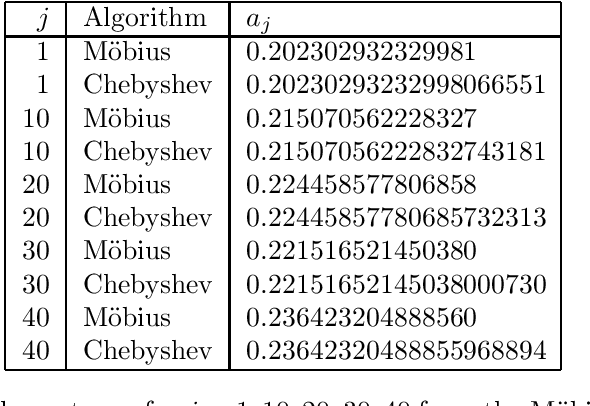•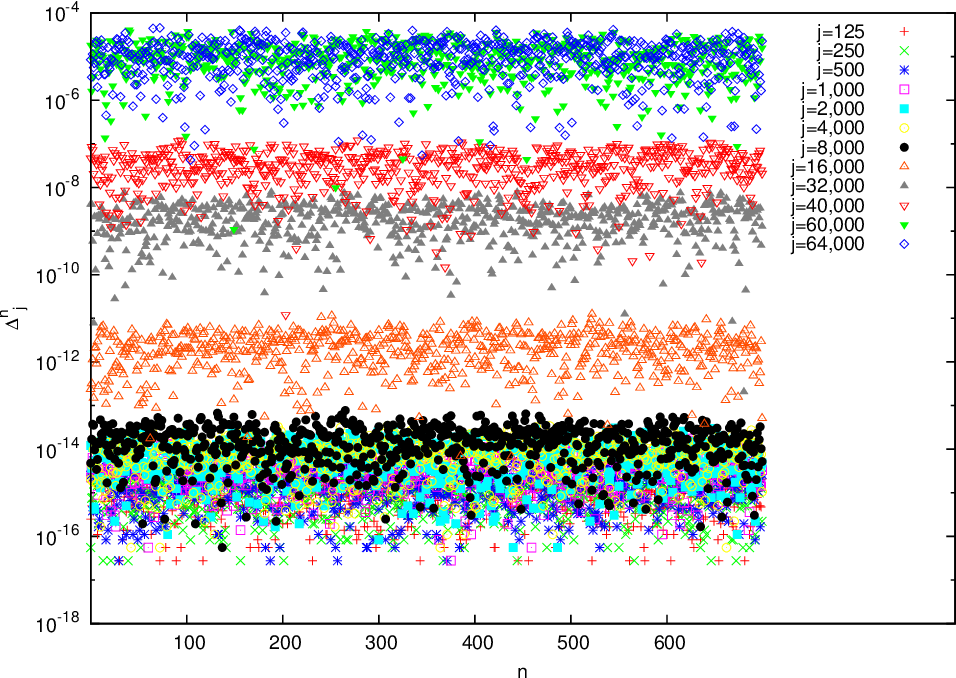•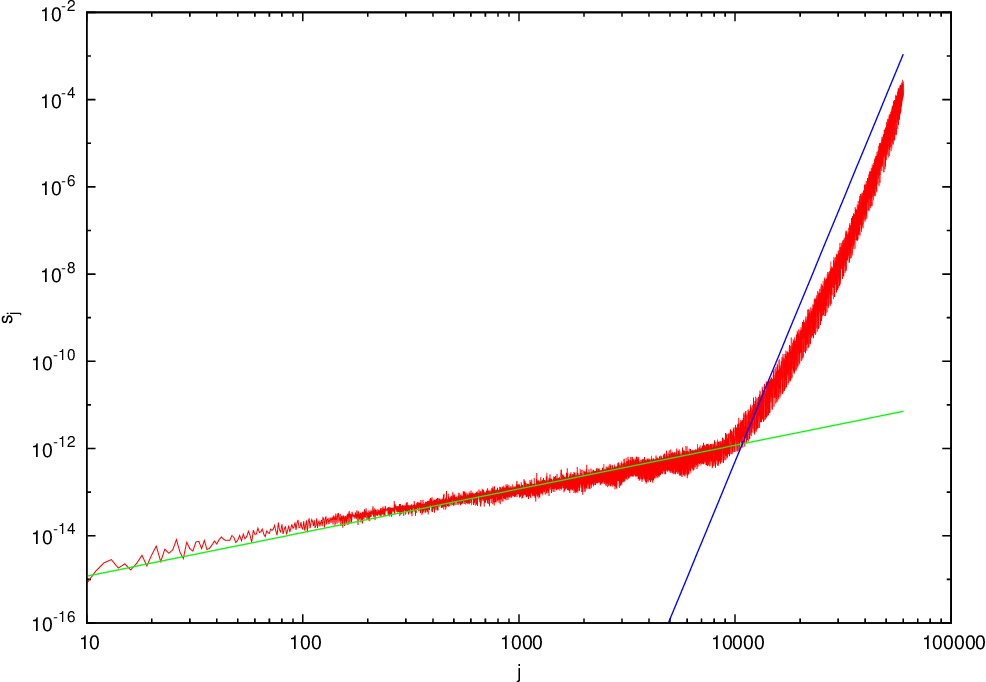2015
2015
• J. Comput. Appl. Math.
• 2015
• Corpus ID: 6024306
Hermann Minkowski introduced a function in 1904 which maps quadratic irrational numbers to rational numbers and this function is… Expand
•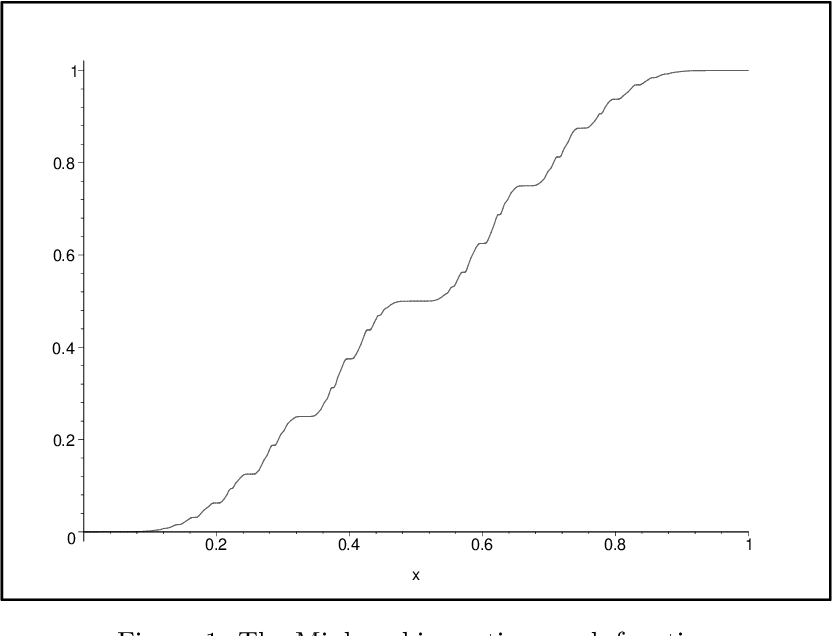•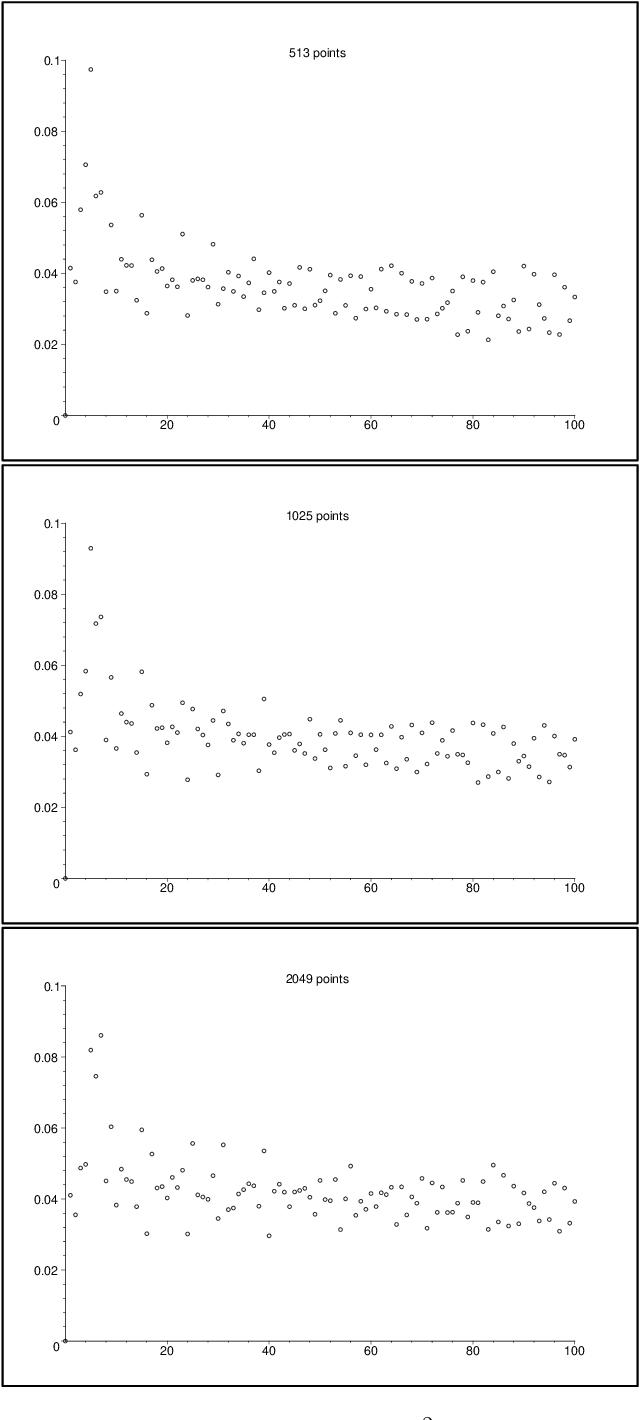•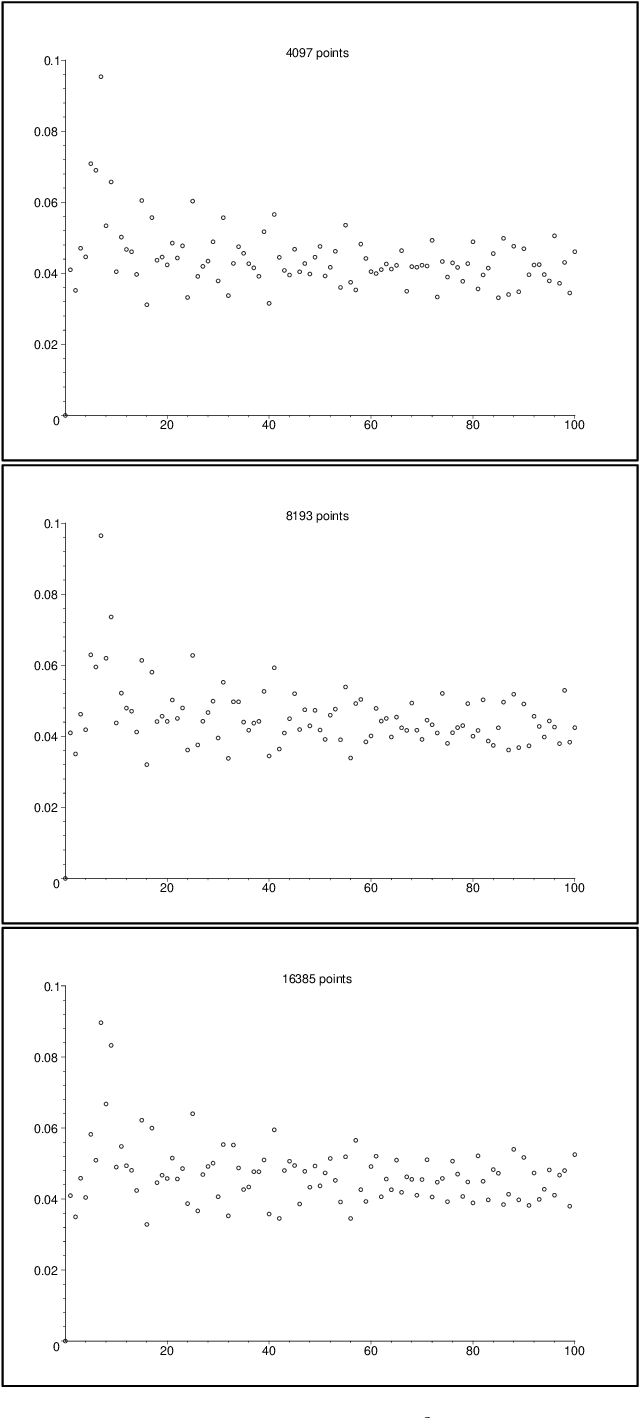•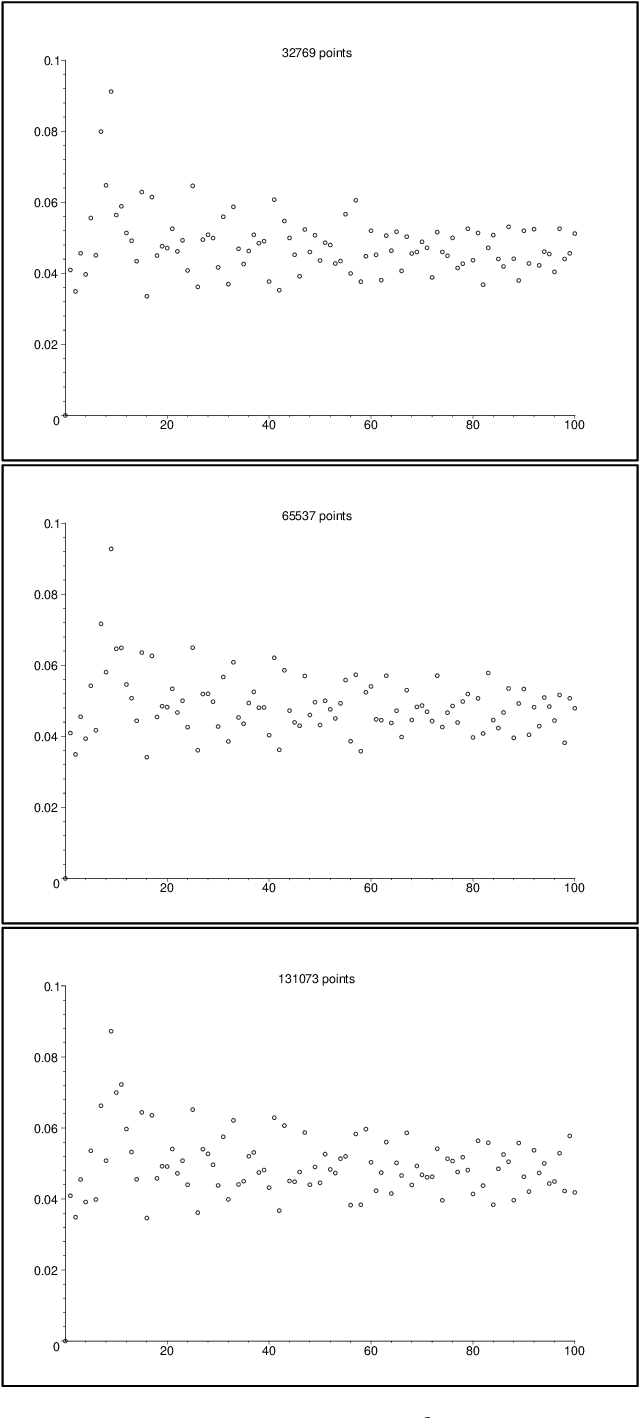•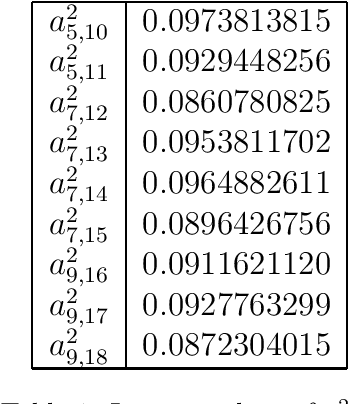2015
2015
Minkowski's question mark function is strictly increasing on \$[0, 1]\$ and hence defines a Stieltjes measure on \$[0, 1]\$. A… Expand
2010
2010
In this paper we investigate the Fourier-Stieltjes coefficients of the Minkowski question mark function. In 1943, R. Salem asked… Expand
•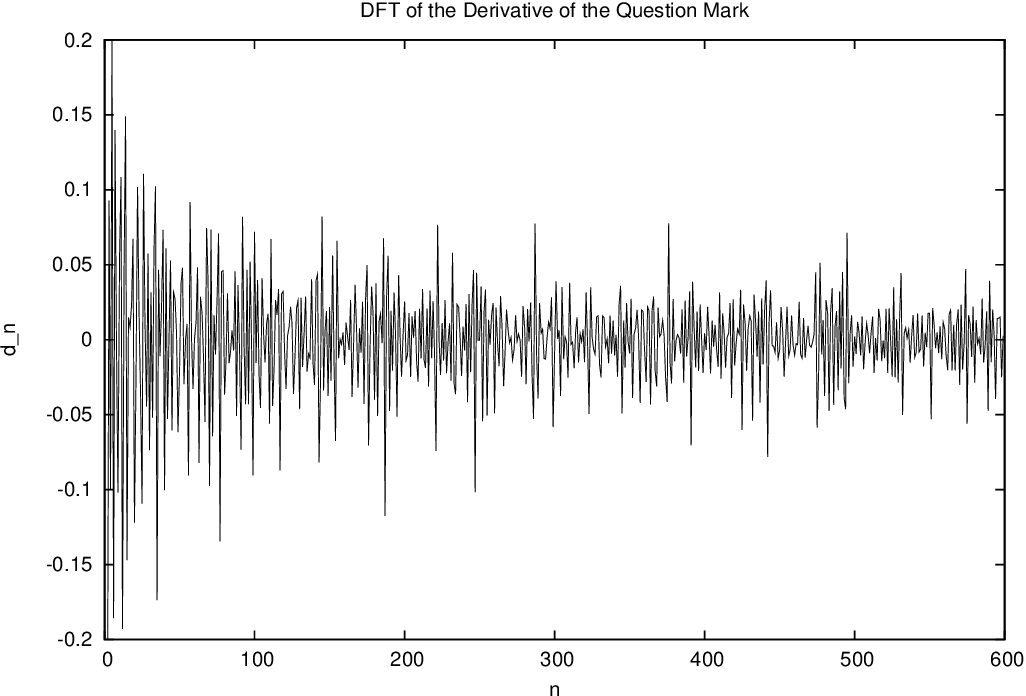•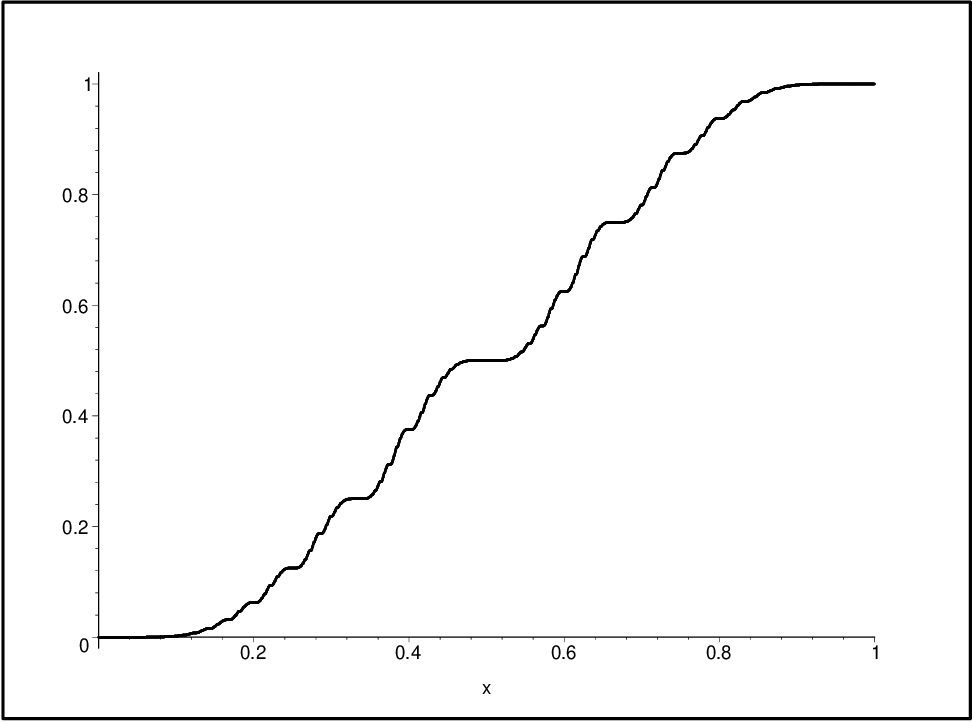•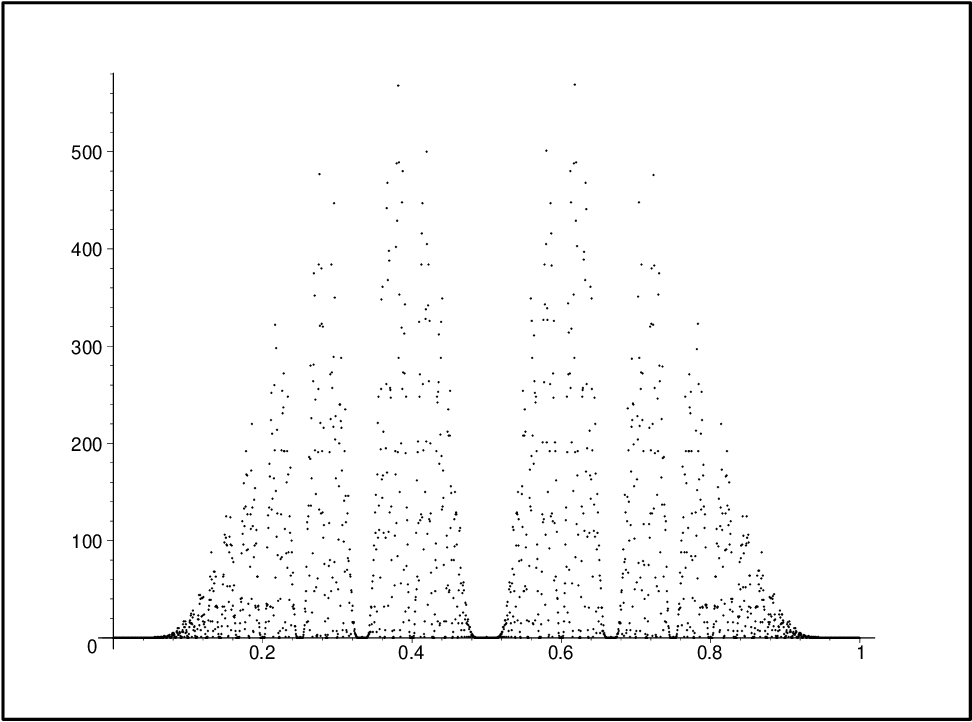•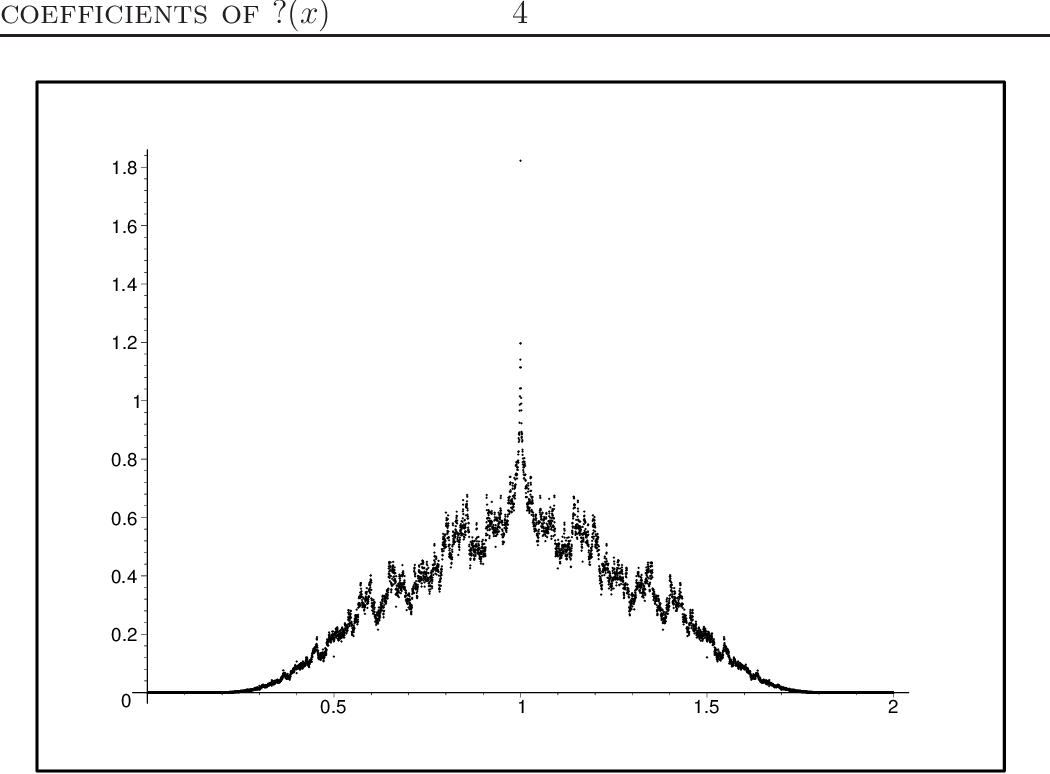•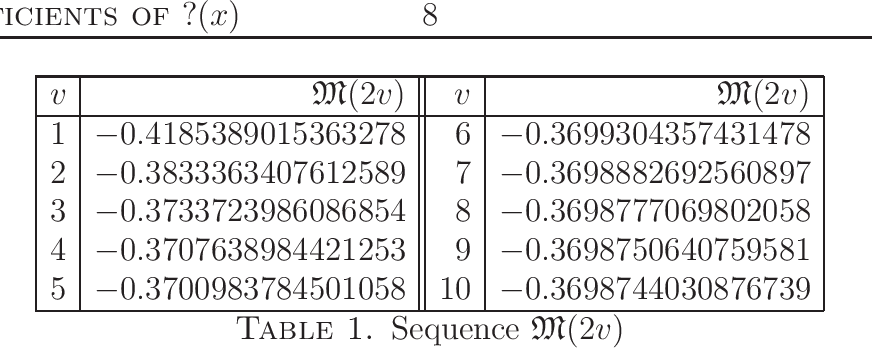2010
2010
The Minkowski question mark function ?( x ) arises as a real distribution of rationals in the Farey tree. We examine the… Expand
•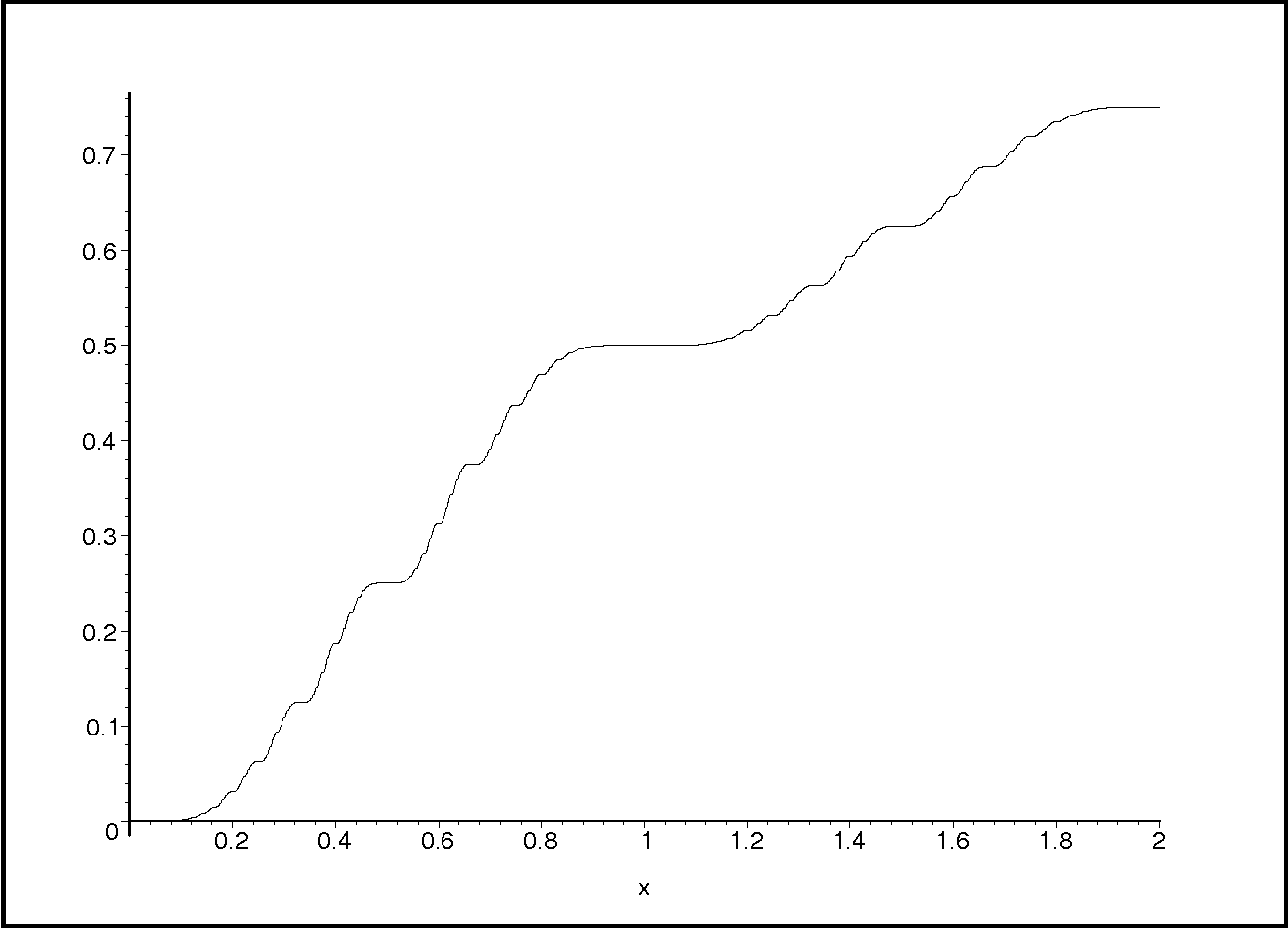2010
2010
Previously, several natural integral transforms of the Minkowski question mark function F(x) were introduced by the author. Each… Expand
•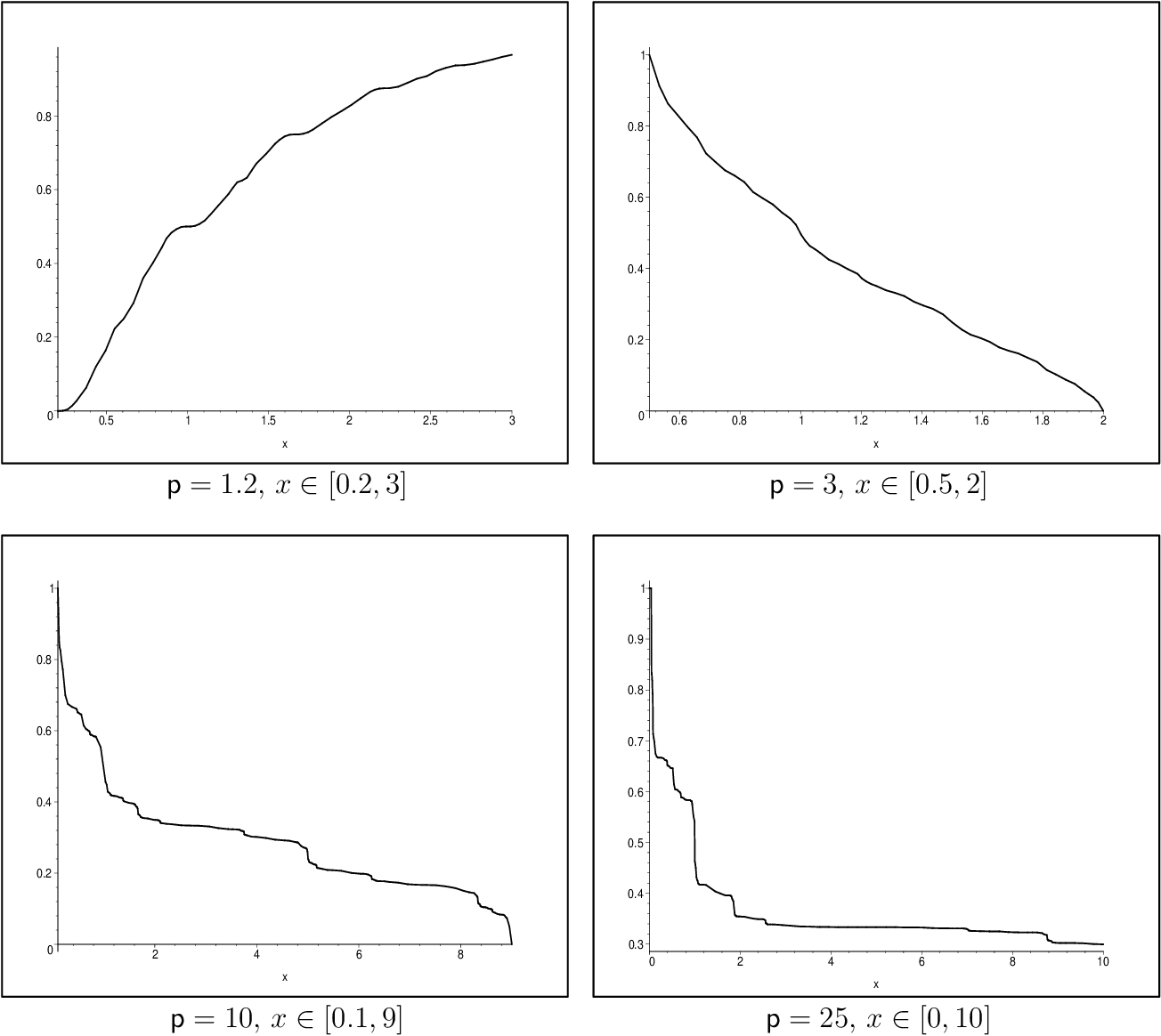2009
2009
• 2009
• Corpus ID: 115176613
Abstract We get necessary and sufficient conditions for the derivative of the Minkowski question mark function ? ( x ) to be… Expand
2008
2008
The Minkowski question mark function F(x) arises as a real distribution function of rationals in the Farey (alias, Stern-Brocot… Expand
2007
2007
• 2007
• Corpus ID: 115156022
Let x = [0; a1, a2, …] be the regular continued fraction expansion of an irrational number x ∈ [0, 1]. For the derivative of the… Expand